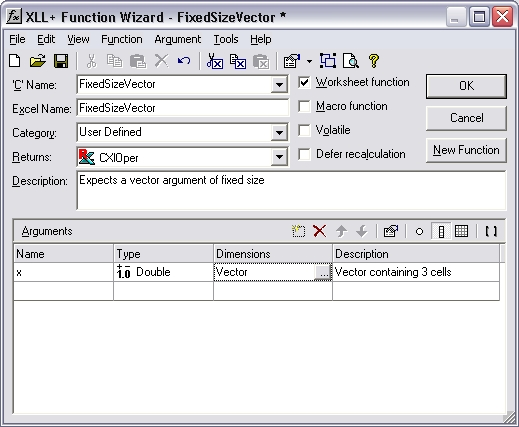HOW TO: How can I make sure that a vector or matrix input is of a fixed size?

Reference: Q0035

• XLL+ for Visual Studio 2005 - 5.0
• XLL+ for Visual Studio .NET - 4.2, 4.3.1, 5.0
• XLL+ for Visual Studio 6 - 3, 4.1, 4.2, 4.3.1, 5.0

HOW TO: How can I make sure that a vector or matrix input is of a fixed size?

Question

My add-in function expects an array of a particular size. Can I use the Function Wizard to specify this constraint?

You can modify your arguments to be bounded input arrays. The XLL+ Function Wizard has a tab which lets you set the bounds of an array, so that they are tied to a constant (or to a named variable). The code which is generated will automatically check that all matrix and vector inputs satisfy the constraints you apply.

If the input is not of the expected size, then an error value is returned, e.g.:

#Error: expected 5 items in x

Example

Problem: constrain a vector input to contain exactly 3 cells.

Steps

1. Create a new function, FixedSizeVector(), using the XLL+ Function Wizard. It takes a single argument x, which is defined as a vector of doubles.2. Select the Dimensions column of the row containing the definition of x, and click on the ... button. (Or use the short-cut Ctrl+E.) This will display the Edit Argument dialog.

Select the Array tab.

In the Bounds box, check the Bounded check-box and set the upper bound (UBound) to be 3, as shown below.3. Press OK to close the dialog.
4. Inspect the changes to the Function Wizard. The Dimensions column now contains the new definition of x: Vector (0 to 3).5. Save the function.

Code

The code generated is shown below:

// Function:    FixedSizeVector
// Purpose:     Expects a vector argument of fixed size

//{{XLP_SRC(FixedSizeVector)
// NOTE - the FunctionWizard will add and remove mapping code here.
//    DO NOT EDIT what you see in these blocks of generated code!
IMPLEMENT_XLLFN2(FixedSizeVector, "RP", "FixedSizeVector", "x",
"User Defined", "Expects a vector argument of fixed size",
"Vector containing 3 cells\000", "B0(0,3)x Vector containing "
"3 cells\0appscope=1\0", 1)

extern "C" __declspec( dllexport )
LPXLOPER FixedSizeVector(const COper* x)
{
XLL_FIX_STATE;
CXlOper xloResult;
BOOL bOk = TRUE;
std::vector<double> vecx;
long ubound_x = 3;
bOk = bOk && x->ReadVectorBounded(vecx, "x", xloResult, 0, ubound_x);
if (!bOk)
return xloResult.Ret();
//}}XLP_SRC

// TODO - Set the value of xloResult
return xloResult.Ret();
}

Explanation

The generated code has been changed from the normal pattern, as shown in bold above.

1. A new variable ubound_x is generated, and is passed as an additional argument to COper::ReadVectorBounded.
3. COper::ReadVectorBounded() checks that the size of the populated region of the input x matches ubound_x, and returns FALSE if it does not.
4. If COper::ReadVectorBounded() fails, the add-in function returns an error value (which is placed in xloResult by COper::ReadVectorBounded().

Result

If x does not contain 3 cells, the following error value is returned:

#Error: expected 3 items in x

and the function does not continue.